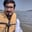Related Tags

general
communitycreator

# What are the types of RNN?Arslan Bajwa

## Recurrent Neural Networks (RNN)

Traditional neural networks mainly have independent input and output layers, which make them inefficient when dealing with sequential data. Hence, a new neural network called Recurrent Neural Network was introduced to store results of previous outputs in the internal memory. These results are then fed into the network as inputs. This allows it to be used in applications like pattern detection, speech and voice recognition, natural language processing, and time series prediction.

RNN has hidden layers that act as memory locations to store the outputs of a layer in a loop.

Recurrent Neural Network

## Types of RNN

The four commonly used types of Recurrent Neural Networks are:

### 1. One-to-One

The simplest type of RNN is One-to-One, which allows a single input and a single output. It has fixed input and output sizes and acts as a traditional neural network. The One-to-One application can be found in Image Classification.

One-to-One

### 2. One-to-Many

One-to-Many is a type of RNN that gives multiple outputs when given a single input. It takes a fixed input size and gives a sequence of data outputs. Its applications can be found in Music Generation and Image Captioning.

One-to-Many

### 3. Many-to-One

Many-to-One is used when a single output is required from multiple input units or a ﻿sequence of them. It takes a sequence of inputs to display a fixed output. Sentiment Analysis is a common example of this type of Recurrent Neural Network.

Many-to-One

### 4. Many-to-Many

Many-to-Many is used to generate a sequence of output data from a sequence of input units.

This type of RNN is further divided into ﻿the following two subcategories:

1. Equal Unit Size: In this case, the number of both the input and output units is the same. A common application can be found in Name-Entity Recognition.

Many-to-Many (Equal)

2. Unequal Unit Size: In this case, inputs and outputs have different numbers of units. Its application can be found in Machine Translation.

Many-to-Many (Unequal)

## Benefits of RNN

Some of the benefits provided by Recurrent Neural Networks are:

1. Processes sequential data

2. Can memorize and store previous results

3. Takes into account both the current and the previous results in the computation of new results

4. Regardless of the increasing size of the input, the model size remains fixed

5. It shares weights to other units across time

## Limitations of RNN

Below are some of the limitations of Recurrent Neural Networks:

1. The computation time is slow as it is recurrent.

2. Unable to process a long sequence of information if using tanh or ReLU activation functions.

3. Cannot process future data in computation of current data.

4. Training is complicated.

5. Exploding Gradient: An exponential increase in model weights occur due to an accumulation of large gradient errors.

6. Vanishing Gradient: The gradients become too small and unable to make significant changes in the model weights.

RELATED TAGS

general
communitycreator

CONTRIBUTORArslan Bajwa
RELATED COURSES

View all Courses

Keep Exploring

Learn in-demand tech skills in half the time